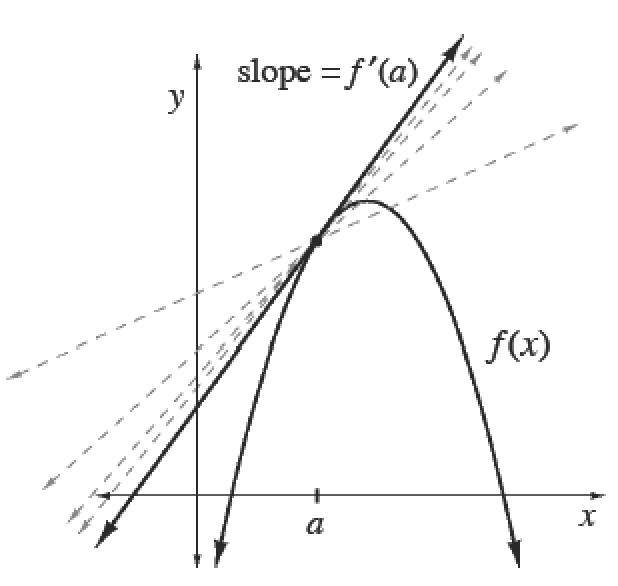### Home > APCALC > Chapter 3 > Lesson 3.2.2 > Problem3-64

3-64.

Using the definition of the derivative as a limit, show that the derivative of $f(x) = \frac { 1 } { x ^ { 2 } }$ is $f^\prime (x) = -\frac { 2 } { x ^ { 3 } }$. That is, show algebraically that the following limit statement is true:

$\lim\limits _ { h \rightarrow 0 } \frac { \frac { 1 } { ( x + h ) ^ { 2 } } - \frac { 1 } { x ^ { 2 } } } { h } = - \frac { 2 } { x ^ { 3 } }$

The Derivative

The slope of a line tangent to at any point $x$ is called the derivative of $f$ at $x$. It is found by taking a limit of the slope of a secant line as $h \rightarrow 0$. The standard form of this type of limit is:

$f^\prime (x) = \lim\limits _ { h \rightarrow 0 } \frac { f ( x + h ) - f ( x ) } { h }$

If the slope (or instantaneous rate of change) at a particular $x$-value is desired, such as at $x = a$, then the notation used is $f^\prime (a)$. This slope can be found by replacing $x$ with $a$.

$f^\prime (a) = \lim\limits _ { h \rightarrow 0 } \frac { f ( a + h ) - f ( a ) } { h }$This is one form of the 'definition of the derivative' (informally known as Hana's Method).

In order to evaluate this limit, we need to find an Algebraic way to cancel out the $h$ in the denominator.

Find a common denominator in the numerator, expand and combine like terms:

$\lim\limits_{h\rightarrow 0}\frac{\frac{x^{2}-(x+h)^{2}}{(x^{2})(x+h)^{2}}}{h}=\lim\limits_{h\rightarrow 0}\frac{x^{2}-(x^{2}+2xh+h^{2})}{h(x^{2})(x+h)^{2}}=\lim\limits_{h\rightarrow 0}\frac{-2xh-h^{2}}{h(x^{2})(x+h)^{2}}$

Factor the numerator, then cancel out the $h$:

$\lim\limits_{h\rightarrow 0}\frac{h(-2x-h)}{h(x^{2})(x+h)^{2}}=\lim\limits_{h\rightarrow 0}\frac{(-2x-h)}{(x^{2})(x+h)^{2}}$

Since there is no longer and $h$ in the denominator, you can evaluate the limit as $h \rightarrow 0$:

$=\lim\limits_{h\rightarrow 0}\frac{(-2x-(0))}{(x^{2})(x+(0))^{2}}=\lim\limits_{h\rightarrow 0}\frac{(-2x)}{(x^{2})(x)^{2}}=-\frac{2}{x^{3}}$